# Cokernel

(diff) ← Older revision | Latest revision (diff) | Newer revision → (diff)

of a morphism in a category

The concept dual to the concept of the kernel of a morphism in a category. In categories of vector spaces, groups, rings, etc. it describes a largest quotient object of an objectthat annihilates the image of a homomorphism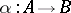.

Let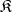be a category with null morphisms. A morphismis called a cokernel of a morphismif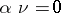and if any morphismsuch that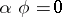can be expressed in unique way as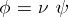. A cokernel of a morphismis denoted by.

If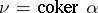and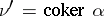thenfor a unique isomorphism.

Conversely, ifandis an isomorphism, thenis a cokernel of. Thus, all cokernels of a morphismform a quotient object of, which is denoted by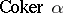. If, thenis a normal epimorphism. The converse need not be true. The cokernel of the zero morphismis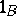. The cokernel of the unit morphismexists if and only ifcontains a zero object.

In a categorywith a zero object, a morphismhas a cokernel if and only ifcontains a co-Cartesian square with respect to the morphismsand. This condition is satisfied, in particular, for any morphism of a right locally small category with a zero object and products.

The co-Cartesian square, or fibred sum or pushout, of two morphisms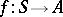,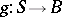is (if it exists) a commutative diagram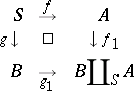such that for any two morphisms,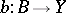such that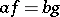there exists a unique morphism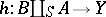for which,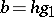.